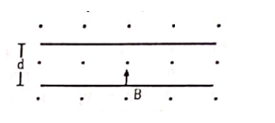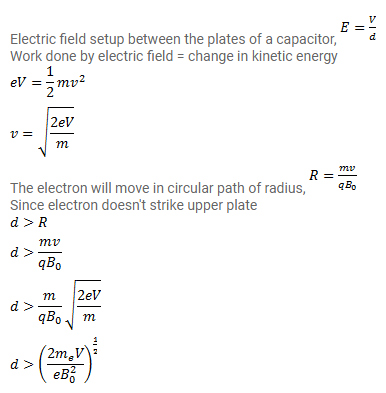# An electron is emitted with negligible speed from the speed

Question:

An electron is emitted with negligible speed from the speed from the negative plate of a parallel plate capacitor charged to a potential difference $V$. The separation between the plates is $d$ and a magnetic field B exists in the space as shown in figure. Show that the electron will fail to strike upper plate if

$d>\left(\frac{2 m_{\theta} V}{e B_{0}^{2}}\right)^{\frac{1}{2}}$Solution: